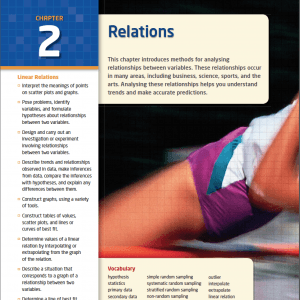Hello Students,

The topics on this page are what I would consider some of the “Big Ideas” of the MPM1D Grade 9 Academic Math Course to assist you in your course review and preparation for the EQAO Grade 9 Assessment of Mathematics which is worth 20% of your final grade. There are four (4) content strands in the course, so I will organize the topics based on strand and only include what I would consider the most important and/or common difficult concepts that all students should review over these last two weeks.

Note that you can find a full course review by clicking here.

# NA – Number Sense and Algebra

## NA1 – Operating With Exponents

### NA1.01 – I can substitute into and evaluate algebraic expressions involving exponents.

Here’s another video, if you want another perspective.

### NA1.03 & NA1.04 – I can … apply the exponent rules for multiplying and dividing monomials, extend the multiplication rule to the power of a power rule … and apply it to simplify expressions involving one and two variables with positive exponents.

Here’s another video for a different perspective.

## NA2 – Manipulating Expressions and Solving Equations

### NA2.07 – I can solve first-degree equations, including equations with fractional coefficients, using a variety of tools and strategies.

#### Solving Simple Equations – One and Two Step Equations

Learn how to solve a simple equation involving two-steps:

Solve:

4m – 6 = 12

#### Solve Two-Step Equations

Learn how to solve a two-step equation:

Solve:

7x – 4 = 10

Solve:

3 + 4m + 5m = 21

#### How to Solve Multi-Step Equations With Variable Terms on Both Sides

Solve:

4y – 13 = -6y + 7

#### How to Solve Equations Involving Distributive Property

Solve:

4(k – 3) = 2 – (2k – 6)

#### How to Solve Equations with One Fraction

Solve:

(1/3)(x – 2) = 5

and

16 = [3(v + 7)]/2

Solve:

[3(z – 5)]/4 = 7

Solve:

3 = [2(n + 7)]/5

#### Solving Equations with One Fraction Application Questions

A trapezoidal backyard has an area of 100 m^2. The front and back widths are 8 m and 12 m, as shown in the diagram.

What is the length of the yard from front to back?

#### Create and Solve Equations from Word Problems

The sum of two consecutive even integers is -134. Find the numbers.

#### Problem Solving Using Equations

The length of Laurie’s rectangular swimming pool is triple its width. The pool covers an area of 192 m^2.

If Laurie swims across the diagonal and back, how far does she travel?

### NA2.08 – I can rearrange formulas involving variables in the first degree, with and without substitution.

Rearrange the following equation for ‘h’

V = p(r^2)h

# LR – Linear Relations

## LR1 – Using Data Management to Investigate Relationships

We see these concepts so much throughout the course, I don’t think much time reviewing is necessary.

## LR2 – Understanding Characteristics of Linear Relations

### LR2.04 – I can compare the properties of direct variation and partial variation in applications, and identify the initial value when described in words, represented as a table, a graph, or an equation.

Watch another direct variation practice problem solution video and more in-depth details about Direct Variation here.

## LR3 – Connecting Various Representations of Linear Relations

### LR3.02 – I can describe a situation that would explain the events illustrated by a given graph of a relationship between two variables.

Recall some of our “Distance from Work” videos:

Practice more distance-time graphs here:

Task 1 [act 1 | act 2 | act 3]
Task 2 [act 2 | act 3]
Task 3 [act 2 | act 3]
Task 4 [act 2 | act 3]
Task 5 [act 2 | act 3]
Task 6 [act 2 | act 3]
Task 7 [act 2 | act 3]
Task 8 [act 2 | act 3]
Task 9 [act 2 | act 3]
Task 10 [act 2 | act 3]

Re-try the gameshow that goes with the tasks here.

# AG – Analytic Geometry

COMING SOON!

## AG3 – Using the Properties of Linear Relations to Solve Problems

### AG3.02 – I can determine the equation of a line when given information about the line such as: the slope and y-intercept; the slope and a point; or two points from the line.

Equations of Lines Given Slope and a Point:

Here’s a practice problem video with a solution for you to try.

Equations of Lines Given Two Points:

# MG – Measurement and Geometry

## MG2 – Solving Problems Involving Perimeter, Area, Surface Area, and Volume

### MG2.03 – I can solve problems involving the areas and perimeters of composite two-dimensional shapes.

Here’s another example video.

Volume

Surface Area

## MPM1D Digital Textbook

### McGraw-Hill Ryerson: Principles of Mathematics, 9While we don’t use the textbook for much more than a question bank, you can access the McGraw-Hill Ryerson Textbook: Principles of Mathematics, 9 Electronic Textbook in PDF Form. Note that the textbook is password protected for only my students due to copyright law.